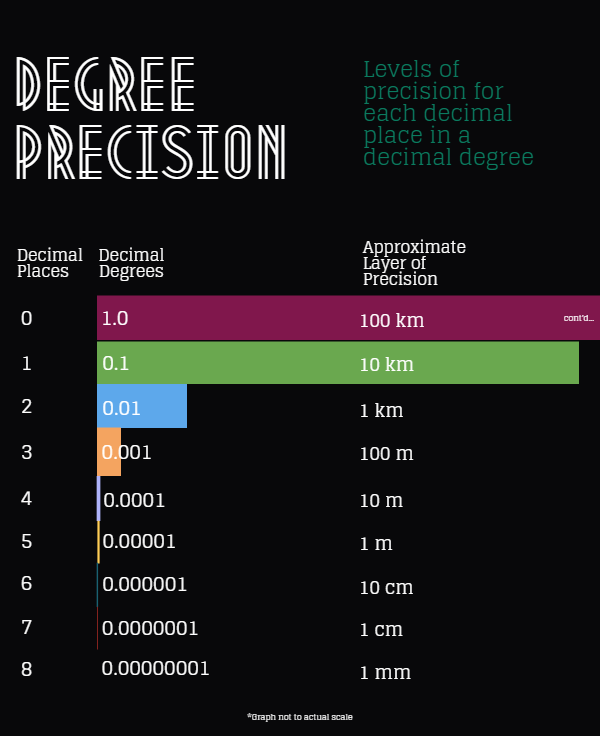# Geocoding Resolution – Ensuring Accuracy and Precision

When geocoding addresses, coordinate precision is not as important as coordinate accuracy. It is a common misconception to confuse high precision decimal degree coordinates with high accuracy. Precision is important, but having a long decimal coordinate for the wrong area could be damaging. It is more important to ensure that the coordinates point to the correct location for the given area. Accurately geocoding an address is very complex. If the address is at all ambiguous or not properly formatted then a geocoding system may incorrectly return a coordinate for a location on the wrong side of town or for a similar looking address in an entirely different state or region.

Some address geocoding systems will return decimal coordinates to the sixth decimal place or more; however, depending on your particular needs, that level of precision may actually prove unnecessary. The degree of precision for most consumer level GPS devices only goes up to the 5th decimal place anyway, which equates to “roughly” one meter of precision. This is “roughly” one meter because the distance can vary depending on how close you are to the either the equator or the poles. The distance will be at its greatest the closer you are to the equator and gradually gets smaller as you move north; however, when dealing with coordinates at this level of precision and above, the difference is mostly negligible for address geocoding.

In the Decimal Degrees wiki page (link below), there is a table that covers the levels of precision for each decimal place in a decimal degree. Below is a similar looking table:Looking at the table above we see that a decimal coordinate with a level of precision past the 6th decimal place would be entirely unnecessary for locating an address. That level of precision would only be necessary under very special circumstances and would require very specialized equipment to use. If a decimal coordinate goes past the 7th or 8th decimal place then the coordinate was most likely calculated and the true level of precision would be unknown. So don’t let a decimal degree coordinate with a high level of precision fool you into thinking that it is more accurate. It is important to always thoroughly test any geocoding system to ensure that it meets your particular needs.

Reference: (https://en.wikipedia.org/wiki/Decimal_degrees)